Discrete Mathematics and Combinatorics Commons™

970 Full-Text Articles 1,129 Authors 104,022 Downloads94 Institutions

All Articles in Discrete Mathematics and Combinatorics

970 full-text articles. Page 36 of 36.

2010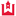Western Kentucky University

An Algorithm To Generate Two-Dimensional Drawings Of Conway Algebraic Knots, Jen-Fu Tung

Masters Theses & Specialist Projects

The problem of finding an efficient algorithm to create a two-dimensional embedding of a knot diagram is not an easy one. Typically, knots with a large number of crossings will not nicely generate two-dimensional drawings. This thesis presents an efficient algorithm to generate a knot and to create a nice two-dimensional embedding of the knot. For the purpose of this thesis a drawing is “nice” if the number of tangles in the diagram consisting of half-twists is minimal. More specifically, the algorithm generates prime, alternating Conway algebraic knots in O(n) time where n is the number of crossings in ...

Minimum Rank Of Skew-Symmetric Matrices Described By A Graph, 2010University of Wyoming

Minimum Rank Of Skew-Symmetric Matrices Described By A Graph, Mary Allison, Elizabeth Bodine, Luz Maria Dealba, Joyati Debnath, Laura Deloss, Colin Garnett, Jason Grout, Leslie Hogben, Bokhee Im, Hana Kim, Reshmi Nair, Olga Pryporova, Kendrick Savage, Bryan Shader, Amy Wangsness Wehe

Mathematics Publications

The minimum (symmetric) rank of a simple graph G over a field F is the smallest possible rank among all symmetric matrices over F whose ijth entry (for i≠j) is nonzero whenever {i,j} is an edge in G and is zero otherwise. The problem of determining minimum (symmetric) rank has been studied extensively. We define the minimum skew rank of a simple graph G to be the smallest possible rank among all skew-symmetric matrices over F whose ijth entry (for i≠j) is nonzero whenever {i,j} is an edge in G and is zero otherwise. We apply ...

2010University of Tennessee, Knoxville

Computational Circle Packing: Geometry And Discrete Analytic Function Theory, Gerald Lee Orick

Doctoral Dissertations

Geometric Circle Packings are of interest not only for their aesthetic appeal but also their relation to discrete analytic function theory. This thesis presents new computational methods which enable additional practical applications for circle packing geometry along with providing a new discrete analytic interpretation of the classical Schwarzian derivative and traditional univalence criterion of classical analytic function theory. To this end I present a new method of computing the maximal packing and solving the circle packing layout problem for a simplicial 2-complex along with additional geometric variants and applications. This thesis also presents a geometric discrete Schwarzian quantity whose value ...

On The Non-Existence Of A Projective (75, 4,12, 5) Set In Pg(3, 7), 2010University of Richmond

On The Non-Existence Of A Projective (75, 4,12, 5) Set In Pg(3, 7), Aaron C.S. Chan, James A. Davis, Jonathan Jedwab

Math and Computer Science Faculty Publications

We show by a combination of theoretical argument and computer search that if a projective (75, 4, 12, 5) set in PG(3, 7) exists then its automorphism group must be trivial. This corresponds to the smallest open case of a coding problem posed by H. Ward in 1998, concerning the possible existence of an infinite family of projective two-weight codes meeting the Griesmer bound.

Minimum Rank Problems, 2010Iowa State University

Minimum Rank Problems, Leslie Hogben

Mathematics Publications

A graph describes the zero–nonzero pattern of a family of matrices, with the type of graph (undirected or directed, simple or allowing loops) determining what type of matrices (symmetric or not necessarily symmetric, diagonal entries free or constrained) are described by the graph. The minimum rank problem of the graph is to determine the minimum among the ranks of the matrices in this family; the determination of maximum nullity is equivalent. This problem has been solved for simple trees [P.M. Nylen, Minimum-rank matrices with prescribed graph, Linear Algebra Appl. 248 (1996) 303–316, C.R. Johnson, A. Leal ...

Maximum Generic Nullity Of A Graph, 2010Iowa State University

Maximum Generic Nullity Of A Graph, Leslie Hogben, Bryan Shader

Mathematics Publications

For a graph G of order n, the maximum nullity of G is defined to be the largest possible nullity over all real symmetric n×n matrices A whose (i,j)th entry (for i≠j) is nonzero whenever {i,j} is an edge in G and is zero otherwise. Maximum nullity and the related parameter minimum rank of the same set of matrices have been studied extensively. A new parameter, maximum generic nullity, is introduced. Maximum generic nullity provides insight into the structure of the null-space of a matrix realizing maximum nullity of a graph. It is shown that ...

Some Implications Of Chu's 10Ψ10 Generalization Of Bailey's 6Ψ6 Summation Formula, 2010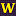West Chester University of Pennsylvania

Some Implications Of Chu's 10Ψ10 Generalization Of Bailey's 6Ψ6 Summation Formula, James Mclaughlin, Andrew Sills

Mathematics

Lucy Slater used Bailey's 6Ã6 summation formula to derive the Bailey pairs she used to construct her famous list of 130 identities of the Rogers-Ramanujan type.

In the present paper we apply the same techniques to Chu's 10Ã10 generalization of Bailey's formula to produce quite general Bailey pairs. Slater's Bailey pairs are then recovered as special limiting cases of these more general pairs.

In re-examining Slater's work, we find that her Bailey pairs are, for the most part, special cases of more general Bailey pairs containing one or more free parameters ...

Recognizing Graph Theoretic Properties With Polynomial Ideals, 2010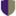University of California - Davis

Recognizing Graph Theoretic Properties With Polynomial Ideals, Jesus A. De Loera, Christopher J. Hillar, Peter N. Malkin, Mohamed Omar

All HMC Faculty Publications and Research

Many hard combinatorial problems can be modeled by a system of polynomial equations. N. Alon coined the term polynomial method to describe the use of nonlinear polynomials when solving combinatorial problems. We continue the exploration of the polynomial method and show how the algorithmic theory of polynomial ideals can be used to detect k-colorability, unique Hamiltonicity, and automorphism rigidity of graphs. Our techniques are diverse and involve Nullstellensatz certificates, linear algebra over finite fields, Gröbner bases, toric algebra, convex programming, and real algebraic geometry.

On The Equivalence Between Real Mutually Unbiased Bases And A Certain Class Of Association Schemes, 2010Worcester Polytechnic Institute

On The Equivalence Between Real Mutually Unbiased Bases And A Certain Class Of Association Schemes, Nicholas Lecompte, William J. Martin, William Owens

Mathematical Sciences Faculty Publications

Mutually unbiased bases (MUBs) in complex vector spaces play several important roles in quantum information theory. At present, even the most elementary questions concerning the maximum number of such bases in a given dimension and their construction remain open. In an attempt to understand the complex case better, some authors have also considered real MUBs, mutually unbiased bases in real vector spaces. The main results of this paper establish an equivalence between sets of real mutually unbiased bases and 4-class cometric association schemes which are both Q-bipartite and Q-antipodal. We then explore the consequences of this equivalence, constructing new cometric ...

Minimum Rank, Maximum Nullity And Zero Forcing Number For Selected Graph Families, 2010University of Puerto Rico, Río Piedras Campus

Minimum Rank, Maximum Nullity And Zero Forcing Number For Selected Graph Families, Edgard Almodovar, Laura Deloss, Leslie Hogben, Kirsten Hogenson, Kaitlyn Murphy, Travis Peters, Camila A. Ramírez

Mathematics Publications

The minimum rank of a simple graph G is defined to be the smallest possible rank over all symmetric real matrices whose ij-th entry (for i≠j) is nonzero whenever {i,j}{i,j} is an edge in G and is zero otherwise. Maximum nullity is taken over the same set of matrices, and the sum of maximum nullity and minimum rank is the order of the graph. The zero forcing number is the minimum size of a zero forcing set of vertices and bounds the maximum nullity from above. This paper defines the graph families ciclos and estrellas and ...

Linear Stochastic State Space Theory In The White Noise Space Setting, 2010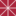Chapman University

Linear Stochastic State Space Theory In The White Noise Space Setting, Daniel Alpay, David Levanony, Ariel Pinhas

Mathematics, Physics, and Computer Science Faculty Articles and Research

We study state space equations within the white noise space setting. A commutative ring of power series in a countable number of variables plays an important role. Transfer functions are rational functions with coefficients in this commutative ring, and are characterized in a number of ways. A major feature in our approach is the observation that key characteristics of a linear, time invariant, stochastic system are determined by the corresponding characteristics associated with the deterministic part of the system, namely its average behavior.

Krein Systems And Canonical Systems On A Finite Interval: Accelerants With A Jump Discontinuity At The Origin And Continuous Potentials, 2010Chapman University

Krein Systems And Canonical Systems On A Finite Interval: Accelerants With A Jump Discontinuity At The Origin And Continuous Potentials, Daniel Alpay, I. Gohberg, M. A. Kaashoek, L. Lerer, A. Sakhnovich

Mathematics, Physics, and Computer Science Faculty Articles and Research

This paper is devoted to connections between accelerants and potentials of Krein systems and of canonical systems of Dirac type, both on a finite interval. It is shown that a continuous potential is always generated by an accelerant, provided the latter is continuous with a possible jump discontinuity at the origin. Moreover, the generating accelerant is uniquely determined by the potential. The results are illustrated on pseudo-exponential potentials. The paper is a continuation of the earlier paper of the authors  dealing with the direct problem for Krein systems.

Discrete-Time Multi-Scale Systems, 2010Chapman University

Discrete-Time Multi-Scale Systems, Daniel Alpay, Mamadou Mboup

Mathematics, Physics, and Computer Science Faculty Articles and Research

We introduce multi-scale filtering by the way of certain double convolution systems. We prove stability theorems for these systems and make connections with function theory in the poly-disc. Finally, we compare the framework developed here with the white noise space framework, within which a similar class of double convolution systems has been defined earlier.

The Maximum Rectilinear Crossing Number Of The Petersen Graph, 2010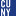CUNY Kingsborough Community College

The Maximum Rectilinear Crossing Number Of The Petersen Graph, Elie Feder, Heiko Harborth, Steven Herzberg, Sheldon Klein

Publications and Research

We prove that the maximum rectilinear crossing number of the Petersen graph is 49. First, we illustrate a picture of the Petersen graph with 49 crossings to prove the lower bound. We then prove that this bound is sharp by carefully analyzing the ten Cs's which occur in the Petersen graph and their properties.

Ergodic And Combinatorial Proofs Of Van Der Waerden's Theorem, 2010Claremont McKenna College

Ergodic And Combinatorial Proofs Of Van Der Waerden's Theorem, Matthew Samuel Rothlisberger

CMC Senior Theses

Followed two different proofs of van der Waerden's theorem. Found that the two proofs yield important information about arithmetic progressions and the theorem. van der Waerden's theorem explains the occurrence of arithmetic progressions which can be used to explain such things as the Bible Code.

Linear Stochastic Systems: A White Noise Approach, 2010Chapman University

Linear Stochastic Systems: A White Noise Approach, Daniel Alpay, David Levanony

Mathematics, Physics, and Computer Science Faculty Articles and Research

Using the white noise setting, in particular the Wick product, the Hermite transform, and the Kondratiev space, we present a new approach to study linear stochastic systems, where randomness is also included in the transfer function. We prove BIBO type stability theorems for these systems, both in the discrete and continuous time cases. We also consider the case of dissipative systems for both discrete and continuous time systems. We further study ℓ1-ℓ2 stability in the discrete time case, and L2-L∞ stability in the continuous time case.

On The Characteristics Of A Class Of Gaussian Processes Within The White Noise Space Setting, 2010Chapman University

On The Characteristics Of A Class Of Gaussian Processes Within The White Noise Space Setting, Daniel Alpay, Haim Attia, David Levanony

Mathematics, Physics, and Computer Science Faculty Articles and Research

Using the white noise space framework, we define a class of stochastic processes which include as a particular case the fractional Brownian motion and its derivative. The covariance functions of these processes are of a special form, studied by Schoenberg, von Neumann and Krein.

Exit Frequency Matrices For Finite Markov Chains, 2009Macalester College

Exit Frequency Matrices For Finite Markov Chains, Andrew Beveridge, László Lovász

Andrew Beveridge

No abstract provided.